The JavaTM Tutorial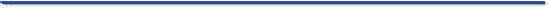Trail: Learning the Java Language
Lesson: Object Basics and Simple Data Objects

## Creating Objects

As you know, a class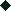provides the blueprint for objects; you create an object from a class. Each of the following statements taken from the `CreateObjectDemo`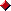program creates an object and assigns it to a variable:
```Point originOne = new Point(23, 94);
Rectangle rectOne = new Rectangle(originOne, 100, 200);
Rectangle rectTwo = new Rectangle(50, 100);
```
The first line creates an object from the `Point`class and the second and third lines each create an object from the `Rectangle`class.

Each statement has the following three parts:

1. Declaration: The code set in bold are all variable declarations that associate a variable name with an object type.
2. Instantiation: The new keyword is a Java operator that creates the object. As discussed below, this is also known as instantiating a class.
3. Initialization: The new operator is followed by a call to a constructor. For example, Point(23, 94) is a call to Point's only constructor. The constructor initializes the new object.
The next three subsections discuss each of these actions in detail:

### Declaring a Variable to Refer to an Object

From the Variablessection in the previous lesson, you learned that to declare a variable, you write:
```type name
```
This notifies the compiler that you will use name to refer to data whose type is type. The Java programming language divides variable types into two main categories: primitive types, and reference types.

Variables of primitive types (byte, short, int, long, char, float, double, or boolean) always hold a primitive value of that same type.

Variables of reference types, however, are slightly more complex. They may be declared in any of the following ways:

• The declared type matches the class of the object:
`MyClass myObject = new MyClass();`

• The declared type is a parent class of the object's class:
`MyParent myObject = new MyClass();`

• The declared type is an interface which the object's class implements:
`MyInterface myObject = new MyClass();`

You can also declare a variable on its own line, such as:

```MyClass myObject;
```
When you use this approach, the value of `myObject` will be automatically set to `null` until an object is actually created and assigned to it. Remember, variable declaration alone does not actually create an object. For that, you need to use the `new` operator, as described in the next section.

A variable in this state, which currently references no object, is said to hold a null reference. If the code in `CreateObjectDemo`had declared its `originOne` variable in this manner, it could be illustrated as follows (variable name, plus reference pointing to nothing):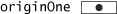A variable of reference type may also hold an object reference, as discussed in the following sections.

### Instantiating a Class

The new operator instantiates a class by allocating memory for a new object.

Note: The phrase "instantiating a class" means the same thing as "creating an object"; you can think of the two as being synonymous. When you create an object, you are creating an instance of a class, therefore "instantiating" a class.

The new operator requires a single, postfix argument: a call to a constructor. The name of the constructor provides the name of the class to instantiate. The constructor initializes the new object.

The new operator returns a reference to the object it created. Often, this reference is assigned to a variable of the appropriate type. If the reference is not assigned to a variable, the object is unreachable after the statement in which the new operator appears finishes executing.

### Initializing an Object

Here's the code for the Point class:
```public class Point {
public int x = 0;
public int y = 0;
//A constructor!
public Point(int x, int y) {
this.x = x;
this.y = y;
}
}
```
This class contains a single constructor. You can recognize a constructor because it has the same name as the class and has no return type. The constructor in the Point class takes two integer arguments, as declared by the code (int x, int y). The following statement provides 23 and 94 as values for those arguments:
```Point originOne = new Point(23, 94);
```
The effect of the previous line of code can be illustrated in the next figure: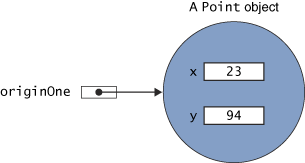Here's the code for the Rectangle class, which contains four constructors:
```public class Rectangle {
public int width = 0;
public int height = 0;
public Point origin;

//Four constructors
public Rectangle() {
origin = new Point(0, 0);
}

public Rectangle(Point p) {
origin = p;
}

public Rectangle(int w, int h) {
this(new Point(0, 0), w, h);
}

public Rectangle(Point p, int w, int h) {
origin = p;
width = w;
height = h;
}

//A method for moving the rectangle
public void move(int x, int y) {
origin.x = x;
origin.y = y;
}

//A method for computing the area of the rectangle
public int area() {
return width * height;
}
}
```
Each constructor lets you provide initial values for different aspects of the rectangle: the origin; the width, and the height; all three; or none. If a class has multiple constructors, they all have the same name but a different number of arguments or different typed arguments. The Java platform differentiates the constructors based on the number and the type of the arguments. When the Java platform encounters the following code, it knows to call the constructor in the Rectangle class that requires a Point argument followed by two integer arguments:
```
Rectangle rectOne = new Rectangle(originOne, 100, 200);
```
This call initializes the rectangle's origin variable to the Point object referred to by originOne. The code also sets width to 100 and height to 200. Now there are two references to the same Point object; an object can have multiple references to it, as shown in the next figure: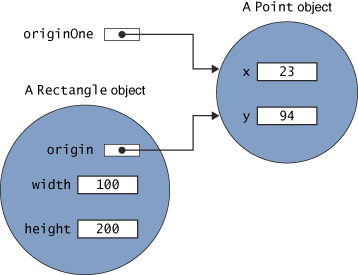The following line of code calls the constructor that requires two integer arguments, which provide the initial values for width and height. If you inspect the code within the constructor, you will see that it creates a new Point object whose x and y values are initialized to 0:
```Rectangle rectTwo = new Rectangle(50, 100);
```
The Rectangle constructor used in the following statement doesn't take any arguments, so it's called a no-argument constructor:
```Rectangle rect = new Rectangle();
```
If a class does not explicitly declare any constructors, the Java platform automatically provides a no-argument constructor, called the default constructor, that does nothing. Thus, all classes have at least one constructor.

This section talked about how to use a constructor.The section Providing Constructors for Your Classesexplains how to write constructors for your classes.